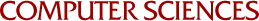Learning in Mathematically-Based Domains: Understanding and Generalizing Obstacle Cancellations

Jude W Shavlik and Gerald F deJong
1989

Mathematical reasoning provides the basis for problem solving and learning in many complex domains. A model for applying explanation-based learning in mathematically-based domains is presented, and an implemented learning system is described. In explanation-based learning, a specific problems solution is generalized into a form that can be later used to solve conceptually similar problems. The presented systems mathematical reasoning processes are guided by the manner in which variables are cancelled in specific problem solutions. Analyzing the cancellation of obstacles  variables that preclude the direct evaluation of the problems unknown  leads to the generalization of the specific solution. Two important general issues in explanation-based learning are also addressed. Namely, generalizing the number of entities in a situation and acquiring efficiently-applicable concepts.